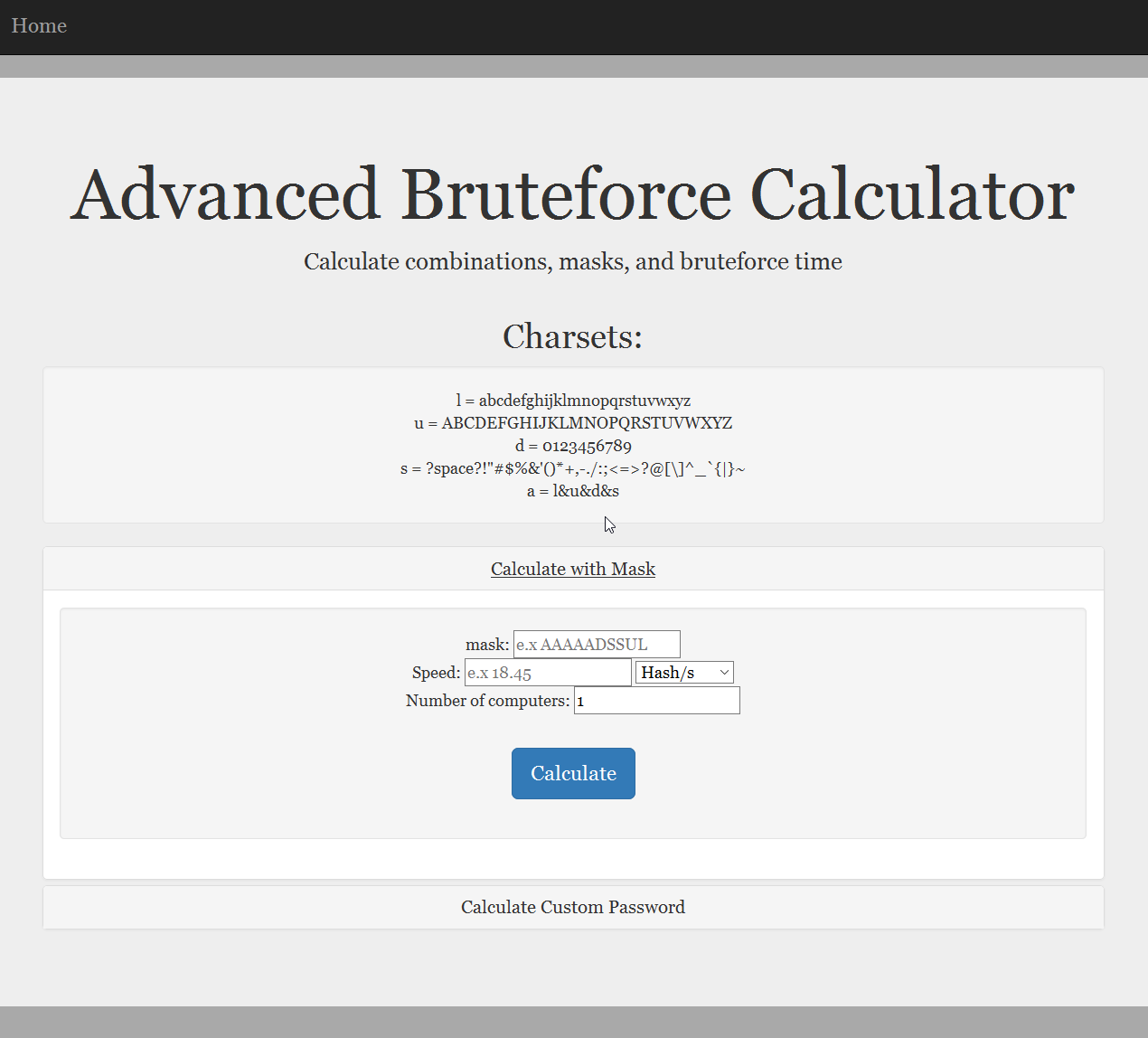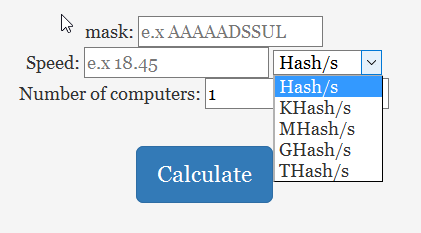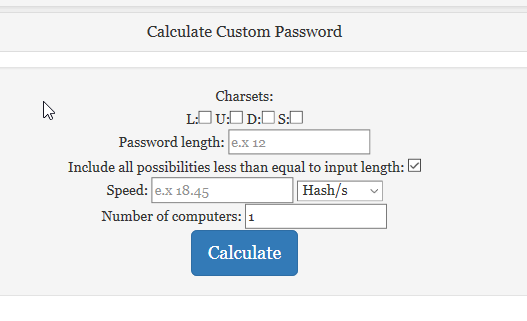# The Bruteforce Calculator!This is the Advanced Bruteforce Calculator.  It’s an online tool to calculate the number of possible combinations based on a mask or custom password attributes, as well as calculate the maximum amount of time needed to bruteforce the password, based on a custom input speed and number of computers. There are two options provided: Calculating using a mask, and calculating using custom password attributes.

This option is for if you wish to specify a custom mask using the predefined charsets provided.

Say, you know that Alice uses a password that is 8 characters long, begins with a number, and ends with a symbol. Can you use this information to help crack her password faster?

Yes.

A mask can be used to reduce bruteforce time. Specifying a mask can reduce the total number possible of combinations in a password if the charsets used, the position of the charsets, and/or the number of re-occurrences of a certain charset is known. Here are the charsets that are currently available:

L = abcdefghijklmnopqrstuvwxyz
U= ABCDEFGHIJKLMNOPQRSTUVWXYZ
D= 0123456789
S= <space>!”#\$%&'()*+,-./:;<=>?@[\]^_`{|}~
A= L&U&D&S

The charsets are based off the charsets in the Cli-based password cracking tool Hashcat.

Now, how do you inform the bruteforce program about the known information? By using a mask! Say, if your password is 5 characters long and contains a digit at the beginning, you can specify it like this: “DAAAA”

Where “D” is the charset containing all base-10 numerical digits, and “A” is the charset containing all uppercase, lowercase, numbers, and symbols (including space).

For Alice, the mask would be: “DAAAAAAS”

A custom mask can be configured in calculating number of combinations and bruteforce time.

## Speed

The “Speed” field is used to input the speed of the attack in order to calculate the bruteforce time.

##The speed can be specified in Hash/s, KHash/s, MHash/s, GHash/s, or THash/s. The number is parsed as a float, therefore decimals are permitted.

## Number of computers

This number is a simple divisor. Setting this number higher than 1 will assume a distributed password cracking scenario where the key space is distributed evenly across multiple machines. The total time and possible combinations will be divided by this number.

## Calculating using custom password attributesThe charsets used in the password can be specified using the four check boxes.